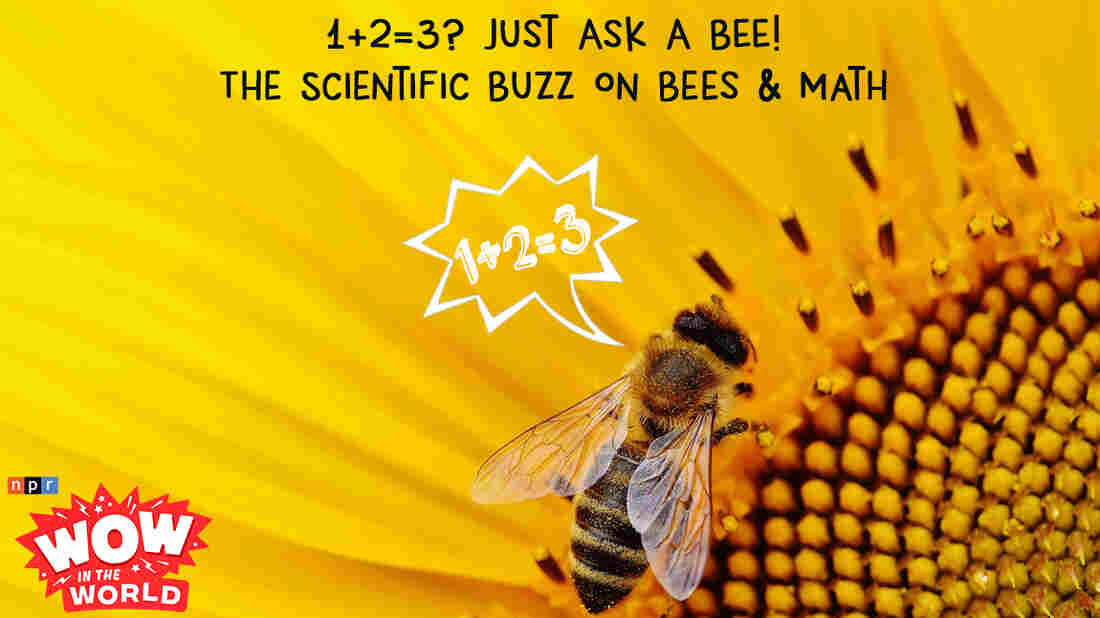1+2=3? Just Ask A BEE! - The Scientific Buzz On Bees & Math : Wow in the World What in the world is a Fibonacci Sequence? Where in the world can you spot one in the wild? And why in the world is that BEE adding and subtracting at the lightening speed of a child?! Join Guy Raz and Mindy as they explore the latest scientific buzz on bees and their newly discovered ability to do...MATH! It's the Who, What, When, Where, Why, How, and Wow in the world of BEE BRAINS!#### 1+2=3? Just Ask A BEE! - The Scientific Buzz On Bees & Math

• `<iframe src="https://www.npr.org/player/embed/699541145/699546565" width="100%" height="290" frameborder="0" scrolling="no" title="NPR embedded audio player">`
• Transcript
1+2=3? Just Ask A BEE! - The Scientific Buzz On Bees & MathNPR

# 1+2=3? Just Ask A BEE! - The Scientific Buzz On Bees & MathNPR

#### 1+2=3? Just Ask A BEE! - The Scientific Buzz On Bees & Math

• `<iframe src="https://www.npr.org/player/embed/699541145/699546565" width="100%" height="290" frameborder="0" scrolling="no" title="NPR embedded audio player">`